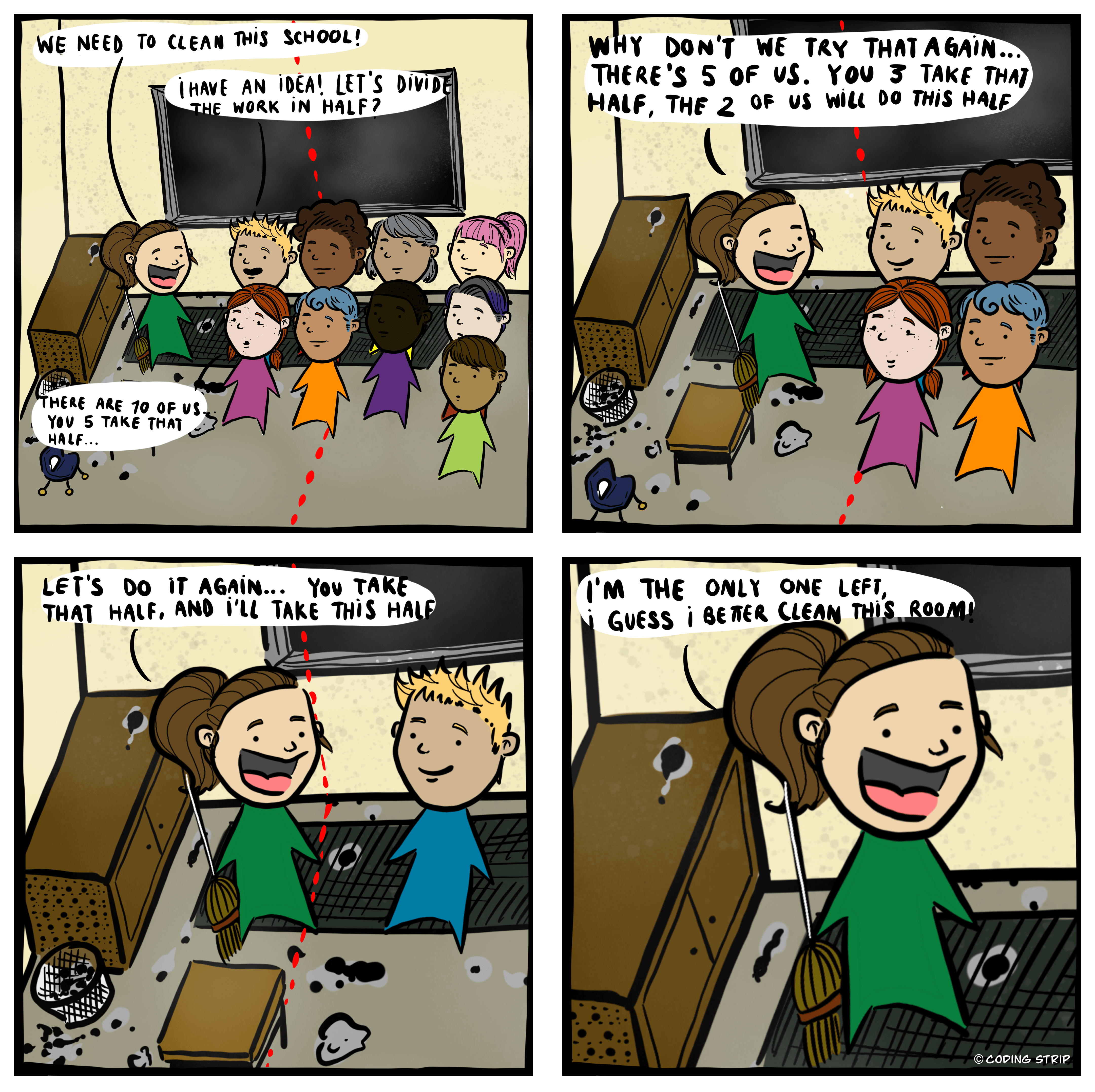# recursion`divideWork(numOfStudents, area) {   if (numOfStudents <= 1) {     clean();   }   else {     students = numOfStudents / 2;     area = area / 2;     divideWork (students, area);     divideWork(numOfStudents - students, area);   }}`